• +91 9971497814
• info@interviewmaterial.com

# Chapter 6- Thermodynamics Interview Questions Answers

### Related Subjects

Question 1 :
Choose the correct answer. A thermodynamic state function is a quantity
(i) used to determine heat changes
(ii) whose value is independent of path
(iii) used to determine pressure volume work
(iv) whose value depends on temperature only.

A thermodynamic state function is a quantity whose value is independent of a path.
Functions like p, V, T etc. depend only on the state of a system and not on the path.
Hence, alternative (ii) is correct.

Question 2 :

For the process to occurunder adiabatic conditions, the correct condition is:

(i) Δ= 0

(ii) Δ= 0

(iii) = 0

(iv) w = 0

A system is said to be under adiabaticconditions if there is no exchange of heat between the system and itssurroundings. Hence, under adiabatic conditions, = 0.

Therefore,alternative (iii) is correct.

Question 3 :

The enthalpies of allelements in their standard states are:

(i) unity

(ii) zero

(iii) < 0

(iv)different for each element

The enthalpy of allelements in their standard state is zero.

Therefore,alternative (ii) is correct.

Question 4 :

ΔUθof combustion of methaneis – X kJ mol–1. The value of ΔHθ is

(i) = ΔUθ

(ii) > ΔUθ

(iii) < ΔUθ

(iv)= 0

Since ΔHθ = ΔUθ + ΔngRT and ΔUθ = –X kJmol–1,

ΔHθ = (–X) + ΔngRT.

ΔHθ < ΔUθ

Therefore,alternative (iii) is correct.

Question 5 :

The enthalpy of combustion of methane,graphite and dihydrogen at 298 K are, –890.3 kJ mol–1 –393.5 kJ mol–1, and –285.8 kJ mol–1 respectively.Enthalpy of formation of CH4(g) will be

(i) –74.8 kJ mol–1            (ii) –52.27 kJ mol–1

(iii) +74.8 kJ mol–1          (iv) +52.26 kJ mol–1.

According to the question,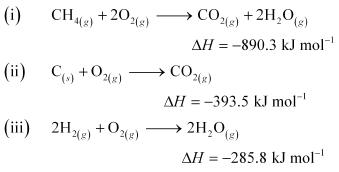Thus, the desired equation is the one thatrepresents the formation of CH4 (g) i.e.,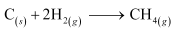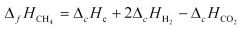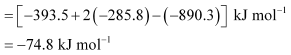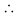Enthalpy of formation of CH4(g= –74.8 kJ mol–1

Hence,alternative (i) is correct.

Question 6 :

A reaction, A + B → C + D + q isfound to have a positive entropy change. The reaction will be

(i) possible at hightemperature

(ii) possible only at lowtemperature

(iii) not possible at anytemperature

(iv)possible at any temperature

For a reaction to be spontaneous, ΔG shouldbe negative.

ΔG = ΔH – TΔS

According to the question,for the given reaction,

ΔS = positive

ΔH = negative (since heat isevolved)

ΔG = negative

Therefore, the reaction isspontaneous at any temperature.

Hence,alternative (iv) is correct.

Question 7 :

Ina process, 701 J of heat is absorbed by a system and 394 J of work is done bythe system. What is the change in internal energy for the process?

According to the first lawof thermodynamics,

ΔU = q + W (i)

Where,

ΔU = change in internal energyfor a process

q = heat

W = work

Given,

q = + 701 J (Since heat is absorbed)

W = –394 J (Since work is done by thesystem)

Substituting the values inexpression (i), we get

ΔU = 701 J + (–394 J)

ΔU = 307 J

Hence,the change in internal energy for the given process is 307 J.

Question 8 :

The reaction of cyanamide, NH2CN(s),with dioxygen was carriedout in a bomb calorimeter, and Î”was found to be â€“742.7kJ molâ€“1at 298 K. Calculate enthalpy change for thereaction at 298 K.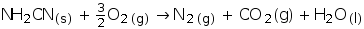Enthalpy change for a reaction (Î”H) is given by theexpression,

Î”H = Î”U + Î”ngRT

Where,

Î”U = change in internalenergy

Î”ng = change in numberof moles

For the given reaction,

Î”ng = âˆ‘ng (products) â€“ âˆ‘ng (reactants)

= (2 â€“ 1.5) moles

Î”ng = 0.5 moles

And,

Î”U = â€“742.7 kJ molâ€“1

T = 298 K

R = 8.314 Ã— 10â€“3 kJ molâ€“1 Kâ€“1

Substituting the values in the expression ofÎ”H:

Î”H = (â€“742.7 kJ molâ€“1) + (0.5 mol) (298 K)(8.314 Ã— 10â€“3 kJ molâ€“1 Kâ€“1)

= â€“742.7 + 1.2

Î”H = â€“741.5 kJ molâ€“1

Question 9 :

Calculate the number of kJ of heat necessaryto raise the temperature of 60.0 g of aluminium from 35°C to 55°C. Molar heatcapacity of Al is 24 J mol–1 K–1.

From the expression of heat (q),

q = m. c. ΔT

Where,

c = molar heat capacity

m = mass of substance

ΔT = change in temperature

Substituting the values in the expressionof q: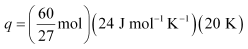q = 1066.7 J

q = 1.07 kJ

Question 10 :

Calculate the enthalpy change on freezing of1.0 mol of water at 10.0°C to ice at –10.0°C. Δfus= 6.03 kJ mol–1 at 0°C.

Cp[H2O(l)] = 75.3 J mol–1 K–1

Cp[H2O(s)] = 36.8 J mol–1 K–1

Total enthalpy changeinvolved in the transformation is the sum of the following changes:

(a) Energy change involvedin the transformation of 1 mol of water at 10°C to 1 mol of water at 0°C.

(b) Energy change involvedin the transformation of 1 mol of water at 0° to 1 mol of ice at 0°C.

(c) Energy change involvedin the transformation of 1 mol of ice at 0°C to 1 mol of ice at –10°C.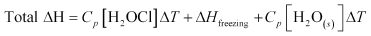= (75.3 J mol–1 K–1) (0 – 10)K + (–6.03 × 103 J mol–1) + (36.8 J mol–1 K–1) (–10 – 0)K

= –753 J mol–1 – 6030 J mol–1 – 368 J mol–1

= –7151 J mol–1

= –7.151 kJ mol–1

Hence,the enthalpy change involved in the transformation is –7.151 kJ mol–1.

Todays Deals### Chapter 6- Thermodynamics Contributorskrishan

Name:
Email:

# Latest News# 9000 interview questions in different categories